Test: Engineering Mathematics & General Aptitude - 2

# Test: Engineering Mathematics & General Aptitude - 2

Test Description

## 20 Questions MCQ Test | Test: Engineering Mathematics & General Aptitude - 2

Test: Engineering Mathematics & General Aptitude - 2 for Electronics and Communication Engineering (ECE) 2023 is part of Electronics and Communication Engineering (ECE) preparation. The Test: Engineering Mathematics & General Aptitude - 2 questions and answers have been prepared according to the Electronics and Communication Engineering (ECE) exam syllabus.The Test: Engineering Mathematics & General Aptitude - 2 MCQs are made for Electronics and Communication Engineering (ECE) 2023 Exam. Find important definitions, questions, notes, meanings, examples, exercises, MCQs and online tests for Test: Engineering Mathematics & General Aptitude - 2 below.
Solutions of Test: Engineering Mathematics & General Aptitude - 2 questions in English are available as part of our course for Electronics and Communication Engineering (ECE) & Test: Engineering Mathematics & General Aptitude - 2 solutions in Hindi for Electronics and Communication Engineering (ECE) course. Download more important topics, notes, lectures and mock test series for Electronics and Communication Engineering (ECE) Exam by signing up for free. Attempt Test: Engineering Mathematics & General Aptitude - 2 | 20 questions in 60 minutes | Mock test for Electronics and Communication Engineering (ECE) preparation | Free important questions MCQ to study for Electronics and Communication Engineering (ECE) Exam | Download free PDF with solutions
 1 Crore+ students have signed up on EduRev. Have you?
Test: Engineering Mathematics & General Aptitude - 2 - Question 1

### Two pipes A and B can fill a cistern in 37*1/2 minutes and 45 minutes respectively. Both pipes are opened. The cistern will be filled in just half an hour, if' the B is turned off after _______ min

Detailed Solution for Test: Engineering Mathematics & General Aptitude - 2 - Question 1

Let B be turned off after x minutes. Then
Part filled by (A+B) in x mm. + part filled by A in (30 -x) mm. = 1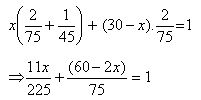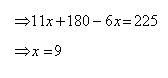Test: Engineering Mathematics & General Aptitude - 2 - Question 2

### A boat can travel with a speed of 13 km/hr in still water. If the speed of the stream is 4 km/hr, find the time taken by the boat to go 68 km downstream.

Detailed Solution for Test: Engineering Mathematics & General Aptitude - 2 - Question 2

A boat can travel with a speed of 13 km/hr in still water. If the speed of the stream is 4 km/hr, find the time taken by the boat to go 68 km downstream.
Speed down stream = (13+4) km/hr = 17 km/hr.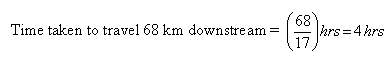Test: Engineering Mathematics & General Aptitude - 2 - Question 3

### What is the probability of getting a sum 9 from two throws of a dice?

Detailed Solution for Test: Engineering Mathematics & General Aptitude - 2 - Question 3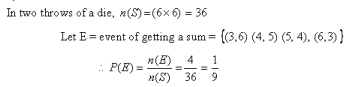Test: Engineering Mathematics & General Aptitude - 2 - Question 4

A Child is looking for his father. He went 90 meters in the east before turning to his right. We went 20 meters before turning to his right again to look for his father at his uncle’s place 30 meters from this point. His father was not there. From there, he went 100 meters to his north before meeting his father in a street. How far did the son meet his father from starting point?

Detailed Solution for Test: Engineering Mathematics & General Aptitude - 2 - Question 4

Clearly, the child moves from A 90 m east words upto B, then turns right and moves 20 m upto C, then turns right and moves 30 m upto D Finally he turns right and moves 100 m upto E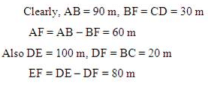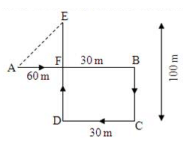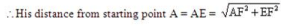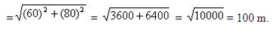Test: Engineering Mathematics & General Aptitude - 2 - Question 5

A and B are brothers C and D are sisters. A’s son is D’s brother. How is B related to C?

Detailed Solution for Test: Engineering Mathematics & General Aptitude - 2 - Question 5

The above problem can be solved from following blood relation tree.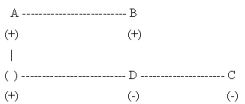Clearly, A is the C’s father. So, the Father’s Brother is uncle, Hence, the answer (D).

Test: Engineering Mathematics & General Aptitude - 2 - Question 6

Choose the box that is similar to the box formed from the given sheet of paper (X).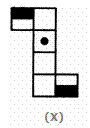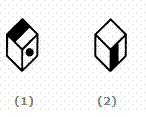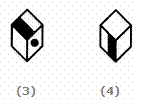Detailed Solution for Test: Engineering Mathematics & General Aptitude - 2 - Question 6

The fig. (X) is similar to Form II. So, when a cube is formed by folding the sheet shown in fig. (X), then the two half-shaded faces lie opposite to each other and one. of the three blank faces appears opposite to the face bearing a dot. Clearly, each one of the four cubes shown in figures (1), (2), (3) and (4) can be formed by folding the sheet shown in fig. (X).

Test: Engineering Mathematics & General Aptitude - 2 - Question 7

Select a suitable figure from the Answer Figures that would replace the question mark (?).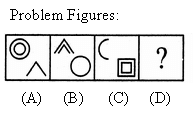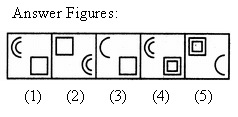Detailed Solution for Test: Engineering Mathematics & General Aptitude - 2 - Question 7

Each one of the upper elements is replaced by an element similar to the lower elernent(s) and each one of the lower elements is replaced by an element similar to the upper element(s).

Test: Engineering Mathematics & General Aptitude - 2 - Question 8

The bar graph given below shows the sales of books (in thousand number) from six branches of a publishing company during two consecutive years 2000 and 2001.
Sales of Books (in thousand numbers) from Six Branches - Bl, B2, B3, B4, B5 and B6 of a publishing Company in 2000 and 2001.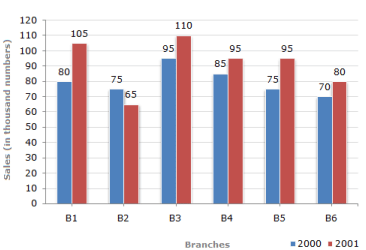Q.

What is the ratio of the total sales of branch B2 for both years to the total sales of branch B4 for both years?

Test: Engineering Mathematics & General Aptitude - 2 - Question 9

The bar graph given below shows the sales of books (in thousand number) from six branches of a publishing company during two consecutive years 2000 and 2001.
Sales of Books (in thousand numbers) from Six Branches - Bl, B2, B3, B4, B5 and B6 of a publishing Company in 2000 and 2001.Q.

Total sales of branch B6 for both the years is what percent of the total sales of branches B3 for both the years

Detailed Solution for Test: Engineering Mathematics & General Aptitude - 2 - Question 9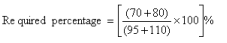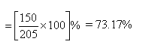Test: Engineering Mathematics & General Aptitude - 2 - Question 10

The bar graph given below shows the sales of books (in thousand number) from six branches of a publishing company during two consecutive years 2000 and 2001.
Sales of Books (in thousand numbers) from Six Branches - Bl, B2, B3, B4, B5 and B6 of a publishing Company in 2000 and 2001.Q.

What percent of the average sales of branches Bl, B2 and B3 in 2001 is the average sales of branches Bl, B3 and B6 in 2000?

Detailed Solution for Test: Engineering Mathematics & General Aptitude - 2 - Question 10

Average sales (in thousand number) of branches Bl, B3 and B6 in 2000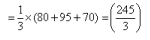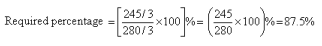Test: Engineering Mathematics & General Aptitude - 2 - Question 11

The values ofk, for which the equations x + y+ z = 1, x+2y + 4z = k and x + 4y + 10z = k2 have at least one solution are

Detailed Solution for Test: Engineering Mathematics & General Aptitude - 2 - Question 11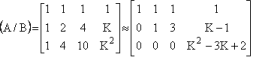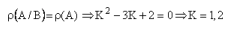Test: Engineering Mathematics & General Aptitude - 2 - Question 12

All eigen values of the matrix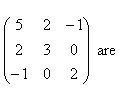Detailed Solution for Test: Engineering Mathematics & General Aptitude - 2 - Question 12

AT=A
Eigen value of real symmetric matrix are real.

Test: Engineering Mathematics & General Aptitude - 2 - Question 13

For the equation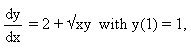then y(1.1) by using Euler’s method is

Detailed Solution for Test: Engineering Mathematics & General Aptitude - 2 - Question 13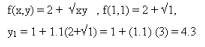Test: Engineering Mathematics & General Aptitude - 2 - Question 14

The function f(x, y) = x2+ bxy + y2 has relative minimum at

Detailed Solution for Test: Engineering Mathematics & General Aptitude - 2 - Question 14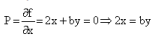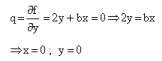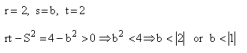Test: Engineering Mathematics & General Aptitude - 2 - Question 15

If f(x,y) = y, g(x,y) = x2 (L is the line segment from (1,1) to (3,5)) then the line integral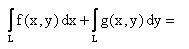Test: Engineering Mathematics & General Aptitude - 2 - Question 16

An article manufactured by a company consists of two parts x & y. In the process of manufacturing the parts x, 9 out of 104 parts may be defective. Similarly 5 out of 100 are likely to be defective in manufacturing the part y. The probability that the assembled product will not be defective is

Detailed Solution for Test: Engineering Mathematics & General Aptitude - 2 - Question 16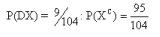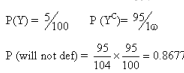Test: Engineering Mathematics & General Aptitude - 2 - Question 17

If U(x, y)=x2-y2 -3x in an analytic function f(z)=U(x, y)+ lV(x, y) then V(x, y) is

Test: Engineering Mathematics & General Aptitude - 2 - Question 18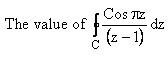taken along the curve C: | z - 1 | = 2 in the positive direction is

Detailed Solution for Test: Engineering Mathematics & General Aptitude - 2 - Question 18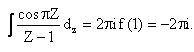Test: Engineering Mathematics & General Aptitude - 2 - Question 19

There are ‘n’ persons sitting in a row, two of them are selected at random the probability that two selected persons are not together is

Detailed Solution for Test: Engineering Mathematics & General Aptitude - 2 - Question 19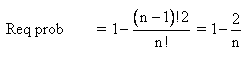Test: Engineering Mathematics & General Aptitude - 2 - Question 20

The minimum of dice are thrown for getting sums at least once greater than 0.95 is ________

Detailed Solution for Test: Engineering Mathematics & General Aptitude - 2 - Question 20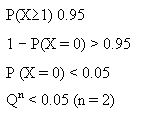Use Code STAYHOME200 and get INR 200 additional OFF Use Coupon Code
Information about Test: Engineering Mathematics & General Aptitude - 2 Page
In this test you can find the Exam questions for Test: Engineering Mathematics & General Aptitude - 2 solved & explained in the simplest way possible. Besides giving Questions and answers for Test: Engineering Mathematics & General Aptitude - 2, EduRev gives you an ample number of Online tests for practice